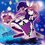# Please Help! I'm Getting Stuck in Inequality

This inequality problem is from Phan Boi Chau High School for the Gifted Grade 10 Selection Test, Nghe An, Vietnam (Yes I'm very bad at inequalities):

Let $$a,b,c$$ be positive reals. Find the minimum value of $$P=\dfrac{a^2}{(a+b)^2}+\dfrac{b^2}{(b+c)^2}+\dfrac{c}{4a}$$.

I've done $$\dfrac{c}{4a}=\dfrac{c^2}{4ac}\geq\dfrac{c^2}{(c+a)^2}$$.

I think the minimum value is $$\dfrac34$$, so the problem is to prove $$\displaystyle \sum_\text{cyc}\dfrac{a^2}{(a+b)^2}\geq\dfrac34$$. But I still can't.

Can you help?Note by Tran Quoc Dat
2 years, 11 months ago

MarkdownAppears as
*italics* or _italics_ italics
**bold** or __bold__ bold
- bulleted- list
• bulleted
• list
1. numbered2. list
1. numbered
2. list
Note: you must add a full line of space before and after lists for them to show up correctly
paragraph 1paragraph 2

paragraph 1

paragraph 2

[example link](https://brilliant.org)example link
> This is a quote
This is a quote
    # I indented these lines
# 4 spaces, and now they show
# up as a code block.

print "hello world"
# I indented these lines
# 4 spaces, and now they show
# up as a code block.

print "hello world"
MathAppears as
Remember to wrap math in $$...$$ or $...$ to ensure proper formatting.
2 \times 3 $$2 \times 3$$
2^{34} $$2^{34}$$
a_{i-1} $$a_{i-1}$$
\frac{2}{3} $$\frac{2}{3}$$
\sqrt{2} $$\sqrt{2}$$
\sum_{i=1}^3 $$\sum_{i=1}^3$$
\sin \theta $$\sin \theta$$
\boxed{123} $$\boxed{123}$$

## Comments

Sort by:

Top Newest

Will Nesbitt's ineq work here?

- 2 years ago

Log in to reply

Continue the solution of @Ameya Daigavane . Using Cauchy-Schwarz $\left(\frac{1}{1 + x}\right)^2 + \left(\frac{1}{1 + y}\right)^2 + \left(\frac{1}{1 + z}\right)^2 \geq\frac{1}{3}\bigg(\frac{1}{x+1}+\frac{1}{y+1}+\frac{1}{z+1}\bigg)^2$ So now we need to have $$f(x,y,z)=\frac{1}{x+1}+\frac{1}{y+1}+\frac{1}{z+1}\geq\frac{3}{2}$$. Without losing generality, we assume that $$x\geq y\geq z$$, then $$f(x,y,z)\geq f(x,y,y)$$.

Now we consider $$f(x,y,y)$$. Since $$x\geq y$$ and $$xy^2=1$$ we can have $$y\leq 1$$ and $$x=\frac{1}{y^2}$$. $f(x,y,y)=\frac{1}{x+1}+\frac{2}{y+1}=\frac{y^2}{y^2+1}+\frac{2}{y+1}=\frac{y^3+3y^2+2}{(y^2+1)(y+1)}$ Now we prove $$f(x,y,y)\geq\frac{3}{2}$$ $\frac{y^3+3y^2+2}{(y^2+1)(y+1)}\geq\frac{3}{2}$ $\Leftrightarrow\frac{2y^3+6y^2+4-3(y^2+1)(y+1)}{2(y+1)(y^2+1)}\geq 0$ $\Leftrightarrow\frac{(1-y)^3}{2(y+1)(y^2+1)}\geq 0 \ (True \ since \ y\leq 1)$ So $$f(x,y,y)\geq\frac{3}{2}\Rightarrow f(x,y,z)\geq\frac{3}{2}$$, therefore $$P\geq\frac{3}{4}$$. The equality holds when $$x=y=z=1$$ or $$a=b=c$$. @Tran Quoc Dat , you can check out this solution, I don't know whether it's ok for grade 9 mathematically specialized students to apply this method

- 2 years, 11 months ago

Log in to reply

univariate solution always cool

- 2 years, 11 months ago

Log in to reply

Is this "mixing of variables"? I think there's some calculus theory behind this method.
Any non calculus approach?

- 2 years, 11 months ago

Log in to reply

Did you mean the $$f(x, y, z) \geq f(x, y, y)$$?

- 2 years, 11 months ago

Log in to reply

Oh no, he changed it, it was $$f(x,y,z) \geq f(x, \sqrt{yz}, \sqrt{yz})$$ I think.

- 2 years, 11 months ago

Log in to reply

Ok. I guess the solution now doesn't use any calculus.

- 2 years, 11 months ago

Log in to reply

I've to change because later on, I found out that actually, $$f(x,y,z)\leq f(x,\sqrt{yz},\sqrt{yz})$$

- 2 years, 11 months ago

Log in to reply

I've tried but looks like there's no non calculus solution to this

- 2 years, 11 months ago

Log in to reply

It's nearly to Olympic more than selection test for grade 10.Huhmmmm

- 2 years, 11 months ago

Log in to reply

After setting, $x = \frac{b}{a}, y = \frac{c}{b}, z = \frac{a}{c}$ this becomes:
For $$x, y, z \in R_+$$ such that $$xyz = 1$$, $\left(\frac{1}{1 + x}\right)^2 + \left(\frac{1}{1 + y}\right)^2 + \left(\frac{1}{1 + z}\right)^2 \geq \frac{3}{4}$

I believe Lagrange Multipliers is the best way here. I'm trying to find a non-calculus approach, and I've posted the question on AoPS as well.

- 2 years, 11 months ago

Log in to reply

This Inequality was used in this problem,Viet Nam TST 2006 inequality problem: PROBLEM

- 2 years, 11 months ago

Log in to reply

×

Problem Loading...

Note Loading...

Set Loading...